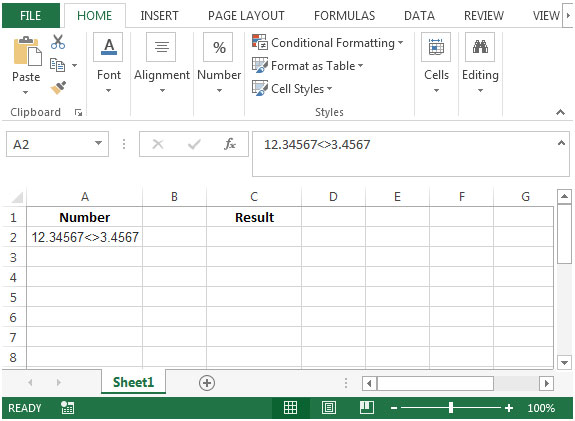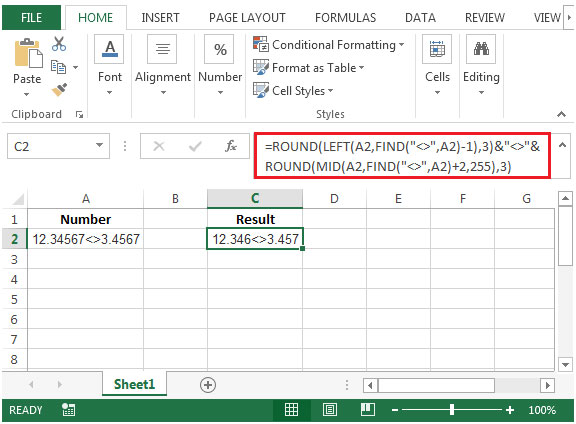# Rounding Numerical Substrings

To find a formula that will retrieve the output by rounding numerical substrings to 3 decimal places, we will use a combination of LEFT, FIND, MID & ROUND functions to retrieve the output.

Let’s say cell A1 contains 12.34567<>3.4567and the output required is 12.346<>3.457

LEFT: Returns the specified number of characters from the start of a text string.

Syntax: =LEFT(text,num_chars)

FIND: Returns the starting position of one text string within another text string. FIND is a case sensitive command.

Syntax: =FIND(find_text,within_text,start_num)

MID: It returns the characters from the middle of a text string, given a starting position and length.

Syntax: =MID(text,start_num,num_chars)

ROUND: Rounds a number to a specified number of digits.

Syntax: =ROUND(number,num_digits)

Let us take an example:

We have a number as 12.34567<>3.4567 in cell A1. We need a formula to show the number to be rounded off by 3 decimal places.• In cell C2, the formula would be
• =ROUND(LEFT(A2,FIND("<>",A2)-1),3)&"<>"&ROUND(MID(A2,FIND("<>",A2)+2,255),3)
• Press enter on your keyboard.
• The function will return the rounded numerical substring.Terms and Conditions of use

The applications/code on this site are distributed as is and without warranties or liability. In no event shall the owner of the copyrights, or the authors of the applications/code be liable for any loss of profit, any problems or any damage resulting from the use or evaluation of the applications/code.